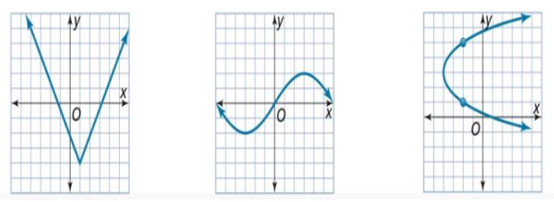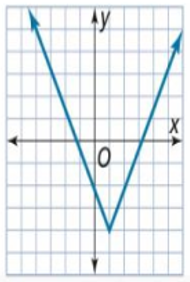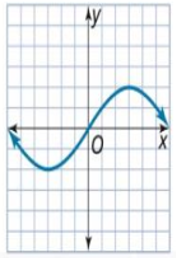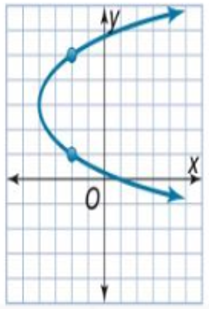Maths-
General
Easy

Question

# Determine whether each graph represents a function ?Hint:

## The correct answer is: not a function

### Step by step solution:We consider the first graph.We can observe that any vertical line drawn on the graph cuts the line at exactly one pointHence, this graph represents a function.The second graph isAgain, we can observe that any vertical line drawn cuts the graph at exactly one point.Hence, this graph is also a function.Finally, consider the third graph.If we draw a vertical line at the origin, that is, the y-axis, we can see that it cuts the graph at two points.Thus, this graph is not a function.

An equation of the form y = f(x) is called a function if there is a unique value of y for every value of x. In other words, every value of x must have one value of y. When we draw a vertical, it cuts x axis at one point, we take that point to be x. If the vertical line cuts the graph at two points, then it gives two values of y for one value of x. This does not represent a function.#### With Turito Foundation.#### Get an Expert Advice From Turito.